Chapter Notes: Circles

# Circles Chapter Notes - Mathematics (Maths) Class 10

 Table of contentsIntroduction to CirclesTheorem 1: Tangent is perpendicular to the radius through the point of contactThe number of Tangents drawn from a given pointLength of a TangentTheorem 2: Lengths of Tangents drawn from an external point## Introduction to Circles

Circle: A circle is a locus of a point that moves in such a way that the distance from that point is always fixed.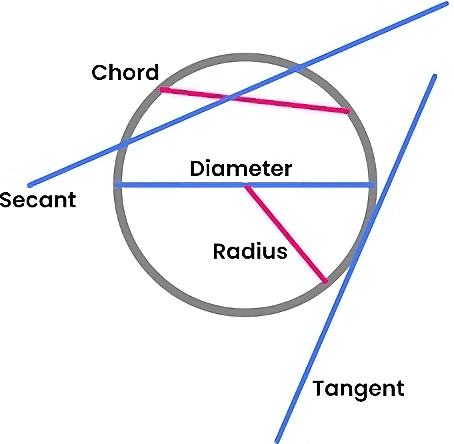Parts of a Circle

• Radius: The constant distance from the centre to the circumference of the circle.
• Secant: A line that intersect the circle at two different points.
• Chord: Any line segment joining the two points on the circumference of the circle.
• Diameter: The longest distance between the two points on the circumference of the circle. It is the longest chord.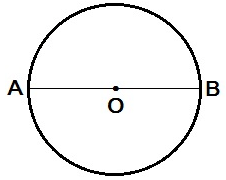Here, AO is the radius of the circle and AB is the diameter of the circle.
• Tangent: A tangent to a circle is a line that touches the circle at exactly one point. For every point on the circle, there is a unique tangent passing through it.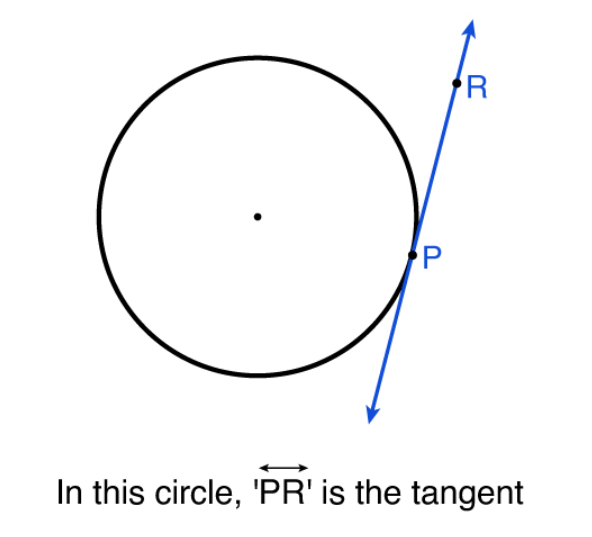Tangent of a Circle

## Theorem 1: Tangent is perpendicular to the radius through the point of contact

The theorem states that “the tangent to the circle at any point is perpendicular to the radius of the circle that passes through the point of contact”.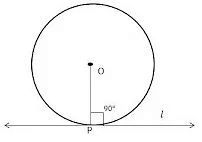In the above diagram l is tangent to the circle at point P. O is the center, Then OP is perpendicular to l.

OP ⊥ l.

Example 1: In the given figure, O is the centre of a circle, AB is a chord and AT is the tangent at A. If ∠AOB = 100°, then calculate ∠BAT.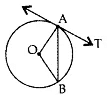Solution: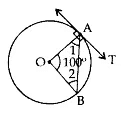∠1 = ∠2

∠1 + ∠2 + 100° = 180°

∠1 + ∠1 = 80°

⇒ 2∠1 = 80°

⇒ ∠1 = 40°

∠1 + ∠BAT = 90°

∠BAT = 90° – 40° = 50°

Question for Chapter Notes: Circles
Try yourself:What is the relationship between the tangent to a circle at any point and the radius of the circle that passes through the point of contact?

## The number of Tangents drawn from a given point

### 1. If the point is in an interior region of the circle

If a point lies inside a circle, any line passing through that point will intersect the circle at two points and is called a secant. Therefore, it is not possible to draw a tangent to a circle that passes through a point inside the circle.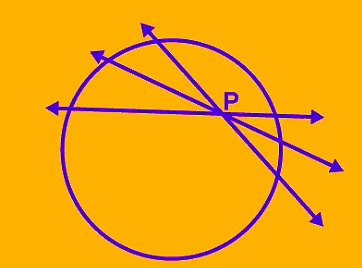### 2. If the point lies on the circle

When a point lies on the circle, there is exactly one tangent to a circle that passes through it.

##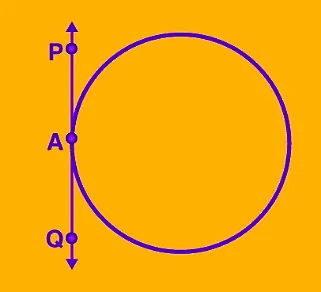### 3. If the point lies outside of the circle

If a point is located outside of a circle, then there exist exactly two tangents that can be drawn to the circle passing through the point.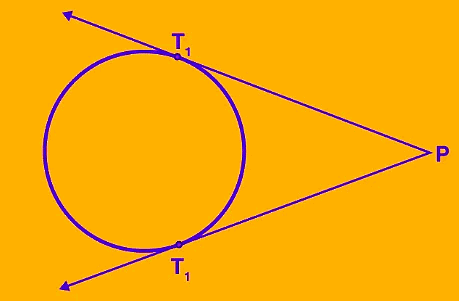## Length of a Tangent

To define the length of a tangent from a point (P) to a circle, we measure the distance from the external point P to the point of tangency I on the circle. This distance is known as the tangent length PI.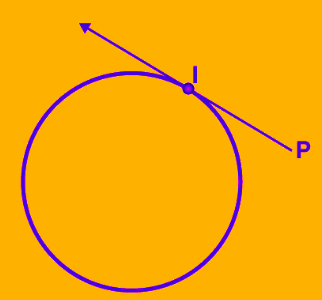## Theorem 2: Lengths of Tangents drawn from an external point

The lengths of tangents drawn from an external point to a circle are equal.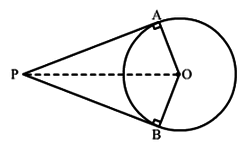In the above diagram, two tangents, AP and BP are drawn from external point P. The theorem states that the length of both tangents will be equal.
AP = BP

Example 2: In the given figure, AB and AC are tangents to the circle with centre o such that ∠BAC = 40°. Then calculate ∠BOC.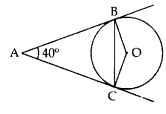Solution: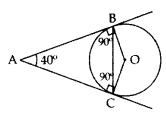AB and AC are tangents
∴ ∠ABO = ∠ACO = 90°
In ABOC,
∠ABO + ∠ACO + ∠BAC + ∠BOC = 360°
90° + 90° + 40° + ∠BOC = 360°
∠BOC = 360 – 220° = 140°

Question for Chapter Notes: Circles
Try yourself:What is the relationship between the tangents drawn from an external point to a circle?

Example 3: In the given figure, PA and PB are tangents to the circle with centre O. If ∠APB = 60°, then calculate ∠OAB.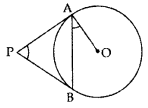Solution: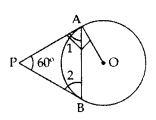∠1 = ∠2
∠1 + ∠2 + ∠APB = 180°
∠1 + ∠1 + 60° = 180°
2∠1 = 180° – 60° = 120°
1

∠1 =  60°
∠1 + ∠OAB = 90°
60° +∠OAB = 90°
∠OAB = 90° – 60° = 30°

Example 4: In the given figure, O is the centre of a circle, PQ is a chord and PT is the tangent at P. If ∠POQ = 70°, then calculate ∠TP.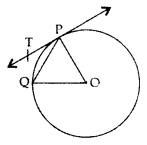Solution: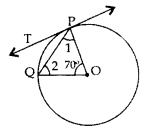∠1 = ∠2
∠1 + ∠2 + 70° = 180°
∠1 + ∠1 = 180° – 70°
2∠1 = 110° ⇒ ∠1 = 55°
∠1 + ∠TPQ = 90°
55° + ∠TPQ = 90°
⇒ ∠TPQ = 90° – 55° = 35°

Example 5: In the given figure, PQ R is a tangent at a point C to a circle with centre O. If AB is a diameter and ∠CAB = 30°. Find ∠PCA.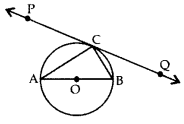Solution:
∠ACB = 90° …[Angle in the semi-circle
In ∆ABC,
∠CAB + ∠ACB + ∠CBA = 180°
30 + 90° + ∠CBA = 180°
∠CBA = 180° – 30° – 90° = 60°
∠PCA = ∠CBA …[Angle in the alternate segment
∴ ∠PCA = 60°

The document Circles Chapter Notes | Mathematics (Maths) Class 10 is a part of the Class 10 Course Mathematics (Maths) Class 10.
All you need of Class 10 at this link: Class 10

## Mathematics (Maths) Class 10

115 videos|478 docs|129 tests

## Mathematics (Maths) Class 10

115 videos|478 docs|129 testsExplore Courses for Class 10 examSignup to see your scores go up within 7 days! Learn & Practice with 1000+ FREE Notes, Videos & Tests.
10M+ students study on EduRev
Track your progress, build streaks, highlight & save important lessons and more!
Related Searches

,

,

,

,

,

,

,

,

,

,

,

,

,

,

,

,

,

,

,

,

,

;